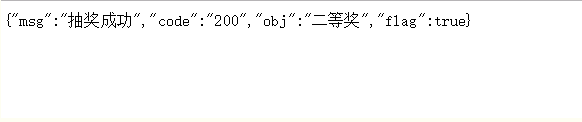# java怎么做出简单的抽奖程序？实例代码展示

BSO 2020-08-31 11:14:52 java常见问答 9069

```public JsonResult luckyDraw()
{
JsonResult jsonResult = new JsonResult("200");
jsonResult.setFlag(true);
jsonResult.setMsg("抽奖成功");
//定义中奖率分母百分之
int probabilityCount = 100;
//最小概率
String min = "min";
//最大概率
String max = "max";
Integer tempInt = 0;
//待中将商品数组
Map < String, Map < String, Integer >> prizeMap = new HashMap < > ();
//获取商品列表
List < Prize > prizeList = prizeDao.findAll();
for (Prize prize: prizeList)
{
Map < String, Integer > oddMap = new HashMap < > ();
//最小概率值
oddMap.put(min, tempInt);
tempInt = tempInt + prize.getPrizeOdd();
//最大概率
oddMap.put(max, tempInt);
prizeMap.put(prize.getId(), oddMap); //(奖品id，最小概率~最大概率)
}
//随机一个数字
int index = (int)(Math.random() * probabilityCount);
Prize prize = null; //中奖商品容器
Set < String > prizeIds = prizeMap.keySet(); //拿到所有奖品id
for (String prizesId: prizeIds)
{
Map < String, Integer > oddMap = prizeMap.get(prizesId); //商品的概率
Integer minNum = oddMap.get(min);
Integer maxNum = oddMap.get(max);
//校验index在那个商品概率中间
if (minNum <= index && maxNum > index)
{
prize = prizeDao.getOne(prizesId);
break;
}
}
if (prize == null)
{
prize = null;
}
jsonResult.setObj(prize.getPrizeName());
return jsonResult;
}```java移位运算符包括哪些?详细实例展示

java工厂模式例子，java实例代码

java如何实现进度条开发的流程?详细实例展示